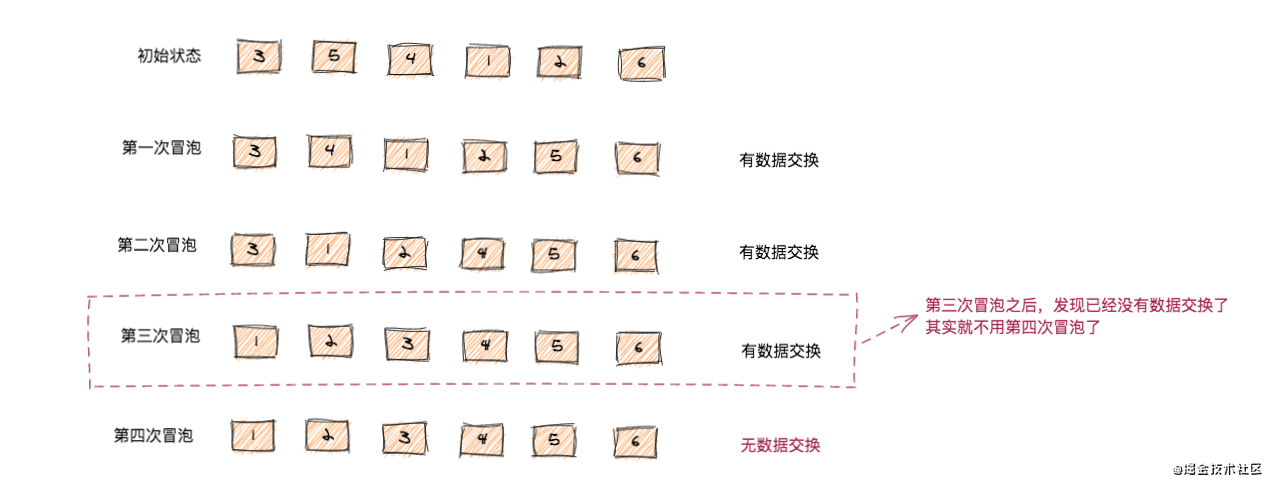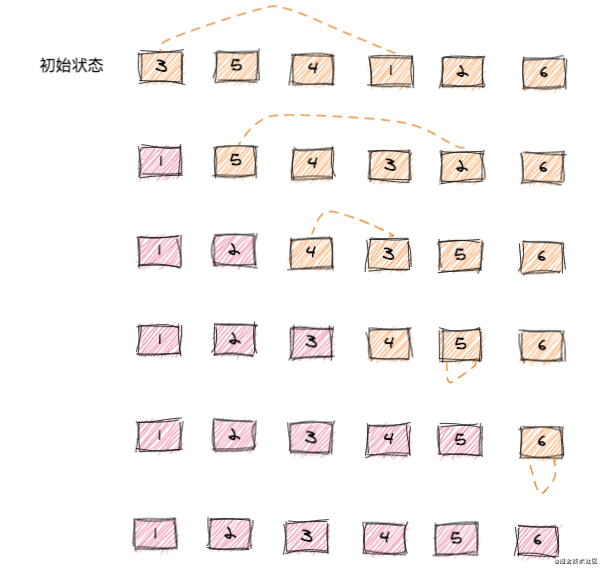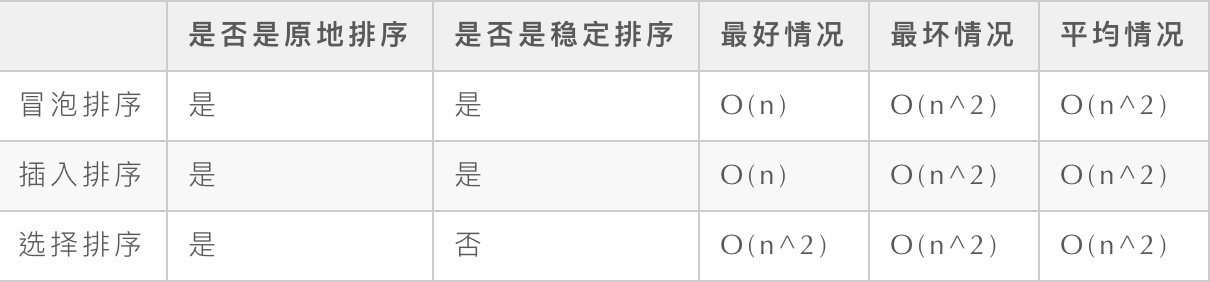你以为只是简单的排序？（一）﻿

﻿

﻿

﻿

﻿

﻿

﻿

﻿

﻿

冒泡排序

﻿

冒泡排序优化

﻿

﻿﻿

func BubbleSort(arr []int) {
flag := false
n := len(arr)
for i := 0; i < n; i++ {
flag = false//如果某一次冒泡，没有出现数据交换，说明已经有序，不用再继续冒泡了
for j := 0; j < n-i-1; j++ {
if arr[j] > arr[j+1] {
tmp := arr[j]
arr[j] = arr[j+1]
arr[j+1] = tmp
flag = true
}
}
if !flag {
break
}
}
for _, v := range arr {
fmt.Printf("%v\t", v)
}
}

﻿

﻿

﻿

﻿

﻿

﻿

插入排序

﻿

插入排序思想

﻿

﻿﻿

func InsertSort(arr []int) {
if len(arr) <= 0 {
fmt.Println("待排数据不合法")
}
n := len(arr)
for i := 1; i < n; i++ {//i是待排区的元素
value := arr[i]
j := i-1
for ; j >= 0; j-- { //j遍历的是已排区的每一个元素
if arr[j] > value {
arr[j+1] = arr[j] //如果满足条件，将前一个值赋给后边这个
} else {
break
}
}
arr[j+1] = value
}
for _, v := range arr {
fmt.Printf("%v\t", v)
}
}

﻿

﻿

﻿

﻿

﻿

选择排序

选择排序思想﻿

func SelectionSort(arr []int) {
n := len(arr)
if n <= 0 {
fmt.Println("待排数据不合法")
}
for i := 0; i < n - 1; i++ {
for j := i+1; j < n ; j++ {
if arr[i] > arr[j] {
arr[i],arr[j] = arr[j], arr[i]
}
}
}
for _, v := range arr {
fmt.Printf("%v\t", v)
}
}

﻿

﻿

﻿

﻿

﻿

三种排序算法对比

﻿

﻿

if arr[j] > arr[j+1] {
tmp := arr[j]
arr[j] = arr[j+1]
arr[j+1] = tmp
flag = true
}

if arr[j] > value {
arr[j+1] = arr[j]
}

﻿

﻿﻿

﻿﻿评论